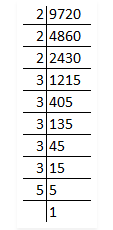# Is 9720 a perfect cube?

Question:

Is 9720 a perfect cube? If not, find the smallest number by which it should be divided to get a perfect cube.

Solution:

First we have to find out the factors by using prime factorisation method.So, prime factors of 9720 = 2 × 2 × 2 × 3 × 3 × 3 × 3 × 3 × 5

Now, grouping the prime factors = (2 × 2 × 2) × (3 × 3 × 3) × 3 × 3 × 5

= 23 × 33 × 3 × 3 × 5

Factors 3 and 4 has no pair.

∴9720 is not a perfect cube.

The smallest number it should be divided to get a perfect cube is 3 × 3 × 5 = 45.

Then,

= 9720 ÷ 45

= 216

Factors of 216 = (2 × 2 × 2) × (3 × 3 × 3)

3√216 = 3√((2 × 2 × 2) × (3 × 3 × 3))

3√(23 × 32)

= 6